# How to add overlay text to Axis cameras

Want to have text overlay in your Axis Camera ?

The camera can display different type of information as shown below.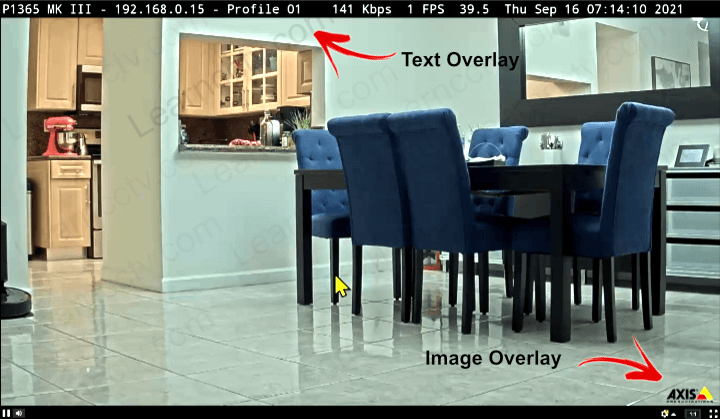As you can see, the camera in the picture displays the following information:

• Camera Name
• Stream Profile
• Bitrate
• Frame rate
• Temperature
• Date and time
• Image

You can use text and variables to display such information.

It's possible to display a image (such as a logo) as long as you upload a .bmp file that with the maximum dimensions of the resolution the stream profile the camera is using. If the resolution is 1920x1080, that will be the image dimensions limitation.

The cameras come with a default Axis logo but you can add other images to the image list and then select any of them to be part of the overlay.

The picture below shows the Overlay Menu.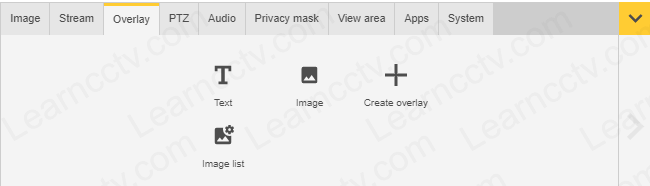To create a new overlay, just click "Create overlay" and enter the text.

You can format the text and the background as you wish. (See picture below).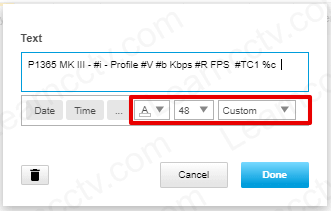As you can see in this example, I typed the camera name and some variables to get the IP Address, Stream Profile, Bitrate, Frame Rate, Temperature and Date/Time.

Click the three dots (...) to check what are the variables that are available.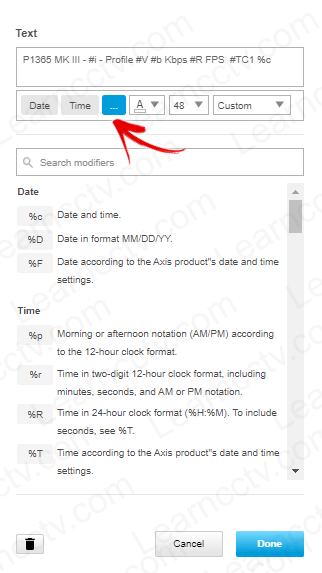You can use a combination of text and variables to display the information the way you need. You can also change the font color and the background.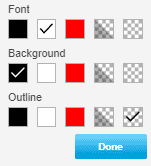## Video: Text Overlay on Axis Cameras

Here's a quick video that shows how the video overlay works (Hosted on YouTube).

## Overlay variables (Modifiers)

Here's a list of modifiers you can use for the camera overlay.

The available modifiers can each be used in various situations. The abbreviations given in brackets for each modifier show which situations the modifier can be used in. These are:

D - Own date formatting

T - Own time formatting

I - Text overlay

EF - Event file name

EN - Event notification via TCP/HTTP

M - Mail subject/message

UF - Folder name for uploads

 Modifier Description (can be used for) %a %a The abbreviated weekday name. (D,I,IP,EF,EN,M,UF). %A %A The full weekday name. (D,I,IP,EF,EN,M,UF). %b %b The abbreviated month name. (D,I,IP,EF,EN,M). %B %B The full month name. (D,I,IP,EF,EN,M,UF). %c %c The date and time. (D,I,IP,EF,EN,M,UF). %C %C The century number (year/100) as a 2-digit integer. (D,I,IP,EF,EN,M,UF). %d %d The day of the month as a decimal number (range 01 to 31). (D,I,IP,EF,EN,M,UF). %D %D Date in format MM/DD/YY. %e %e As for %d, but a leading zero is replaced by a blank space. (D,I,IP,EF,EN,M,UF). %f %f The 1/100 second fraction. (D,I,IP,EF,EN,M,UF). %F %F The date in the format as specified in the Date & Time settings. (I,IP,EF,EN,M,UF). %g %g As for %G, but without the century, i.e., with a 2-digit year (00-99). (D,I,IP,EF,EN,M,UF). %G %G The ISO 8601 year with century as a decimal number. The 4-digit year corresponding to the ISO week number (see %V). This has the same format and value as %y, except that if the ISO week number belongs to the previous or next year, then that year is used instead. (D,I,IP,EF,EN,M,UF). %h %h Equivalent to %b. (D,I,IP,EF,EN,M,UF). %H %H The hour as a decimal number, using the 24-hour clock (range 00 to 23). (T,I,IP,EF,EN,M,UF). %I %I The hour as a decimal number, using the 12-hour clock (range 01 to 12). (T,I,IP,EF,EN,M,UF). %j %j The day of the year as a decimal number (range 001 to 366). (T,I,IP,EF,EN,M,UF). %k %k The hour (24-hour clock) as a decimal number (range 0 to 23); single digits are preceded by a blank space. (See also %H.) (T,I,IP,EF,EN,M,UF). %l %l The hour (12-hour clock) as a decimal number (range 1 to 12); single digits are preceded by a blank. (See also %I.)(T,I,IP,EF,EN,M,UF).
 %m %m The month as a decimal number (range 01 to 12). (D,I,IP,EF,EN,M,UF). %M %M The minute as a decimal number (range 00 to 59). (T,I,IP,EF,EN,M,UF). %p %p AM or PM, according to the given time value, or the corresponding strings for the current locale. Noon is treated as PM and midnight as AM. (T,I,IP,EF,EN,M,UF). %r %r The time in a.m. or p.m. notation. (T,I,IP,EF,EN,M,UF). %R %R The time in 24-hour notation (%H:%M). For a version including seconds, see %T. (T,I,IP,EF,EN,M,UF) Note: Will not work on all FTP servers. %s %s The number of seconds since the Epoch, i.e., since 1970-01-01 00:00:00 UTC. (T,I,IP,EF,EN,M,UF). %S %S The second as a decimal number (range 00 to 61). (T,I,IP,EF,EN,M,UF). %T %T The time as specified in the Date & Time settings. (I,IP,EN,M,UF) Note: Will not work on all FTP servers. %u %u The day of the week as a decimal, range 1 to 7, Monday = 1. See also %W. (D,I,IP,EF,EN,M,UF). %U %U The week number of the current year as a decimal number, range 00 to 53, starting with the first Sunday as the first day of week 01. See also %V and %W. (D,I,IP,EF,EN,M,UF). %v Adds support for 1000 Milliseconds Time Overlay %V %V The ISO 8601:1988 week number of the current year as a decimal number, in the range 01 to 53, where week 1 is the first week that has at least 4 days in the current year, and with Monday as the first day of the week. See also %U, %v and %W. (D,I,IP,EF,EN,M,UF). %w %w The day of the week as a decimal, in the range 0 to 6. Sunday = 0. See also %u. (D,I,IP,EF,EN,M,UF). %W %W The week number of the current year as a decimal number, in the range 00 to 53, starting with the first Monday as the first day of week 01. (D,I,IP,EF,EN,M,UF). %X %X The time representation without the date. (D,I,IP,EN,M,UF). %y %y The year as a decimal number without the century (range 00 to 99). (D,I,IP,EF,EN,M,UF). %Y %Y The year as a decimal number, including the century. (D,I,IP,EF,EN,M,UF).
 %z %z Zoom coordinates (1–19999). %Z %Z Zoom magnification in one-decimal format.
 %% %% The literal % character. (All). #b #b The bit rate in Kbit/s (with no decimals). (I,IP,UF). #B #B The bit rate in Mbit/s (with two decimals) (I,IP,UF). #c #c The compression rate (Stream > General > Compression).
 #e #e The action performed by the Action Rule, for example Send Notification. (EF,EN,M,UF).
 #F #F The frame size in Kbit/s. #D #D Displays a text on top of a video stream image when the Action Rule is triggered. (I,EF,EN,M). #i #i The IP address. (I,IP,EF,EN,M,UF).